# MATLAB--数字图像处理 Hough变换

+关注继续查看

## 前言

Hough变换是1962年由Hough提出来的，用于检测图像中直线、圆、抛物线、椭圆等形状能够用一定函数关系描述的曲线。

## Hough变换原理之基础一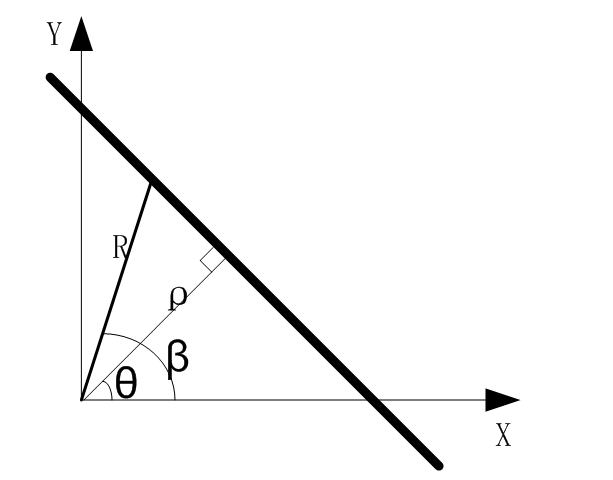L: y=ax+b

R×cos(β-θ)=ρ

R×cosβcosθ+R×sinβsinθ=ρ

x×cosθ+y×sinθ=ρ

## Hough变换原理之基础二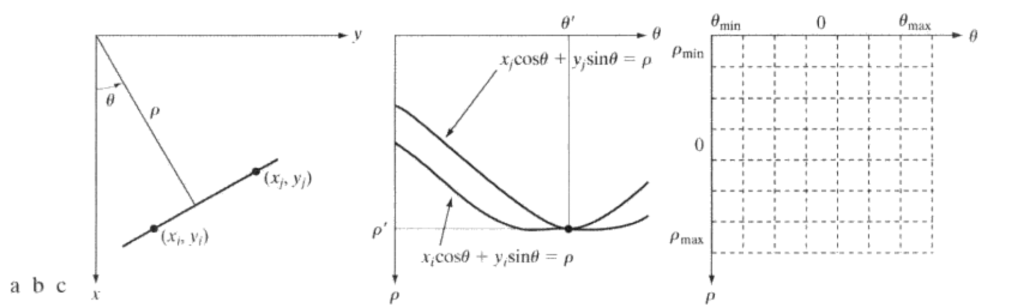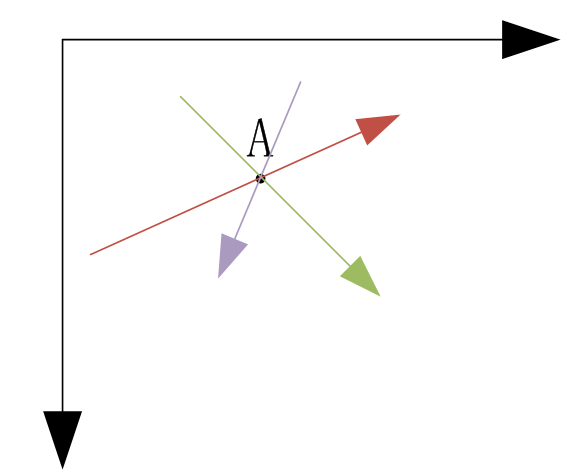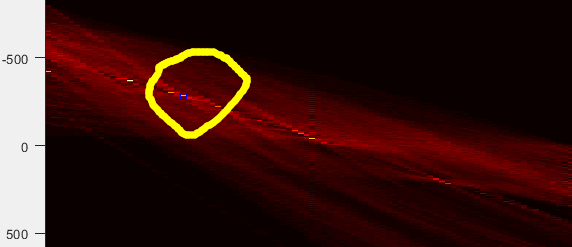## Hough变换原理之基础三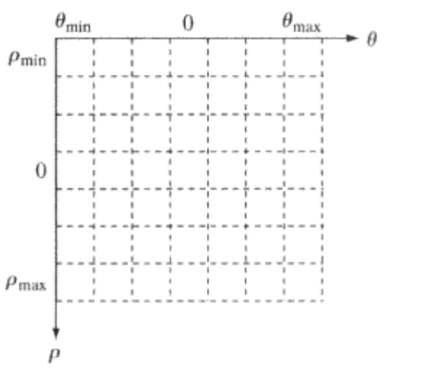## Hough变换原理总结

1. 极坐标与直角坐标系变换
2. 得出每个点在极坐标系的曲线（或直线）
3. 在极坐标系绘画出所有曲线，利用累加的方法寻找需要的点
4. 找到该点，得出（ρ，θ）、直线的起始点、终点坐标

## 利用Hought变换寻找图像中的最长直线

 I = imread('k3.png');
I=rgb2gray(I);
figure;
subplot(131), imshow(I);

% Rotate the image
rotI = I;

% Create a binary image
BW = edge(rotI, 'canny');
subplot(133), imshow(BW);

% Create the hough transform using the binary image
[H, T, R] = hough(BW);
figure, imshow(H, [], 'XData', T, 'YData', R, 'InitialMagnification', 'fit');
xlabel('\theta'), ylabel('\rho');
axis on, axis normal, hold on;
colormap(gca, hot);

% Find peaks in the hough transform of the image
P = houghpeaks(H, 1);
x = T(P(:,2));
y = R(P(:,1));
plot(x, y, 's', 'color', 'blue');

% Find lines and plot them
lines = houghlines(BW, T, R, P, 'FillGap', 5, 'MinLength', 7);
figure, imshow(rotI), hold on;
max_len = 0;
for k = 1 : length(lines)  % here length(lines)=12
xy = [lines(k).point1; lines(k).point2];
% Determine the endpoints of the longest line segment
len = norm(lines(k).point1 - lines(k).point2); % distance between point1 and point2
if ( len > max_len )
max_len = len;
xy_long = xy;
xy = [lines(k).point1; lines(k).point2];
end
end
plot(xy(:, 1), xy(:, 2), 'LineWidth', 2, 'Color', 'green');

% Plot beginnings and ends of lines
plot(xy(1,1), xy(1,2), 'x', 'LineWidth', 2, 'Color', 'yellow');
plot(xy(2,1), xy(2,2), 'x', 'LineWidth', 2, 'Color', 'red');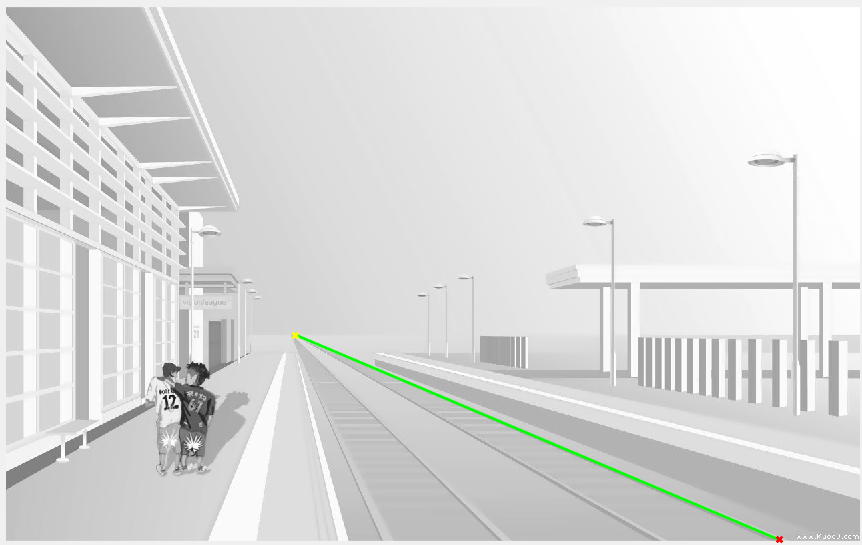|
7月前
|

185 0
|
7月前
|

MATLAB--数字图像处理 cumsum()
MATLAB--数字图像处理 cumsum()
42 0
|
7月前
|

MATLAB--数字图像处理 sort()函数
MATLAB--数字图像处理 sort()函数
59 0
|
7月前
|

MATLAB--数字图像处理 bwlabel()函数
MATLAB--数字图像处理 bwlabel()函数
123 0